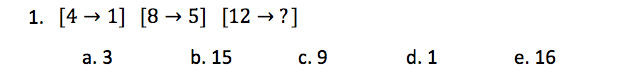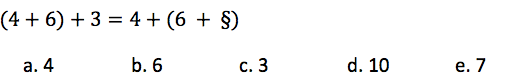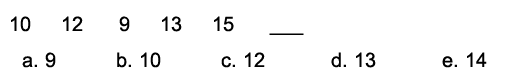Start Now with 100 Free Questions
View MoreHide Information

# CogAT Quantitative Battery

This section of the CogAT test measures a student’s comprehension of scenarios that require problem solving as well as quantitative reasoning. Students are expected to identify relationships between numerical items, while trying to discover rule(s) that govern how a question can be solved. Students are required to use abstract reasoning and relational abilities and relational comparisons to solve the equations presented to them. The quantitative battery consists of number analogies, number puzzles, and number series.

## Number Analogies

This section requires that students rely upon the same thought processes as they use when solving Picture Analogies. Still, instead of verbal concepts, students must identify relationships between quantitative concepts.

Sample Question: In this section, you are given two pairs of numbers and then a third number without its pair. The first two pairs of numbers are related in some way. Figure out what the relationship is between the numbers within each of the pairs. Choose an answer that creates a third pair of numbers that are related to each other in the same way the first two pairs are related.Answer- C (subtract 3 from the first number)

## Number Puzzles

Students will be required to solve equations by choosing the answer choice that allows the amounts on either side of the equal side to amount to the same number. They must use basic operations such as addition, subtraction, division or multiplication to do so.

Sample Question: In this section, you will be given a mathematical equation. Choose the answer that should replace the ? or the symbol.## Number Series

For this type of question, students are presented with a series of images of beads or an abacus. The student must determine the string of beads that comes next in the sequence. They will solve for this question based on the pattern that presents itself.

Sample Question: Look at the numbers in the row below. There is a rule that governs the order in which the numbers occur in the series. Figure out the rule and then choose the number that comes next from the answer choices below.3 Responses

Rashataha2005

it was nice that you put sample s and explained i would reccomend explaining more specifacly

RyanR

Hi,

We do offer brief explanations for our sample problems so that you can figure out why the answer choice is correct. If you would like more details about how to approach these types of problems, then I would recommend taking a look at our online Cogat tutoring!

All the best,
Ryan

jahnavianche

This is ok